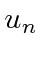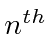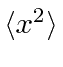## Sample Test Problems

1. A 1D harmonic oscillator is in a linear combination of the energy eigenstatesFind the expected value of.2. Assumingrepresents the1D harmonic oscillator energy eigenstate, calculate.3. Evaluate the uncertainty'' infor the 1D HO ground statewhere. Similarly, evaluate the uncertainty infor the ground state. What is the product?
Its easy to see thateither from the integral or using operators. I'll use operators to compute the rest.4. Use the commutator relation betweenandto derive. Now show thatis the lowering operator for the harmonic oscillator energy.
5. At, a one dimensional harmonic oscillator is in the state. Calculate the expected value ofas a function of time.
6. At, a harmonic oscillator is in a linear combination of theandstates.Findandas a function of time.
7. A 1D harmonic oscillator is in a linear combination of the energy eigenstatesFind.
Jim Branson 2013-04-22